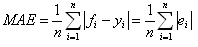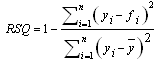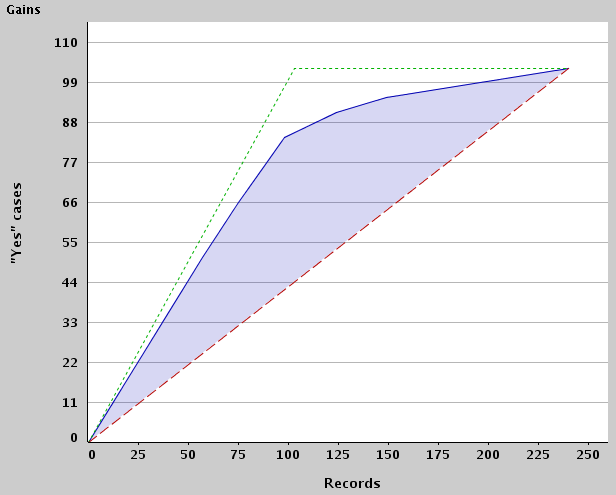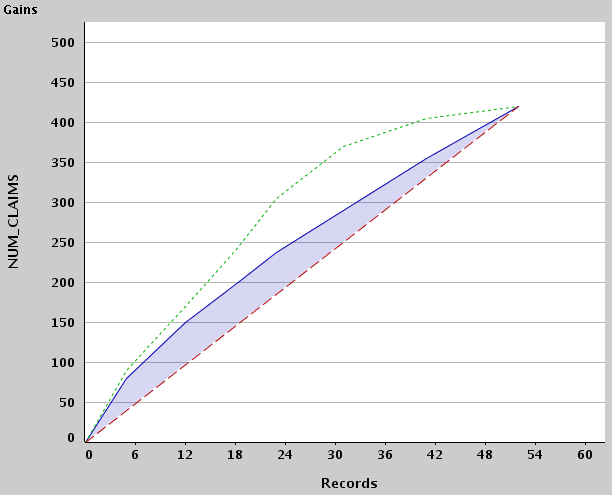This document gives an overview of components that can hold further information to explain PMML models. This can be done by, but is not limited to, visualizing the respective information.PMML 4.4.1 - Model Explanation
 PMML4.4 Menu Home Changes XML Schema Conformance Interoperability General Structure Field Scope Header Data Dictionary Mining Schema Transformations Statistics Taxomony Targets Output Functions Built-in Functions Model Verification Model Explanation Multiple Models Anomaly Detection Models Association Rules Baseline Models Bayesian Network Cluster Models Gaussian Process General Regression k-Nearest Neighbors Naive Bayes Neural Network Regression Ruleset Scorecard Sequences Text Models Time Series Trees Vector Machine

## PMML 4.4.1 - Model Explanation

The following elements can be used: While univariate statistics and partitions are included in specific parts of the models, all the remaining elements are combined into a single element ModelExplanation.
```<xs:element name="ModelExplanation">
<xs:complexType>
<xs:sequence>
<xs:element ref="Extension" minOccurs="0" maxOccurs="unbounded"/>
<xs:choice>
<xs:element ref="PredictiveModelQuality" minOccurs="0" maxOccurs="unbounded"/>
<xs:element ref="ClusteringModelQuality" minOccurs="0" maxOccurs="unbounded"/>
</xs:choice>
<xs:element ref="Correlations" minOccurs="0"/>
</xs:sequence>
</xs:complexType>
</xs:element>
```

### Univariate Statistics

To provide univariate statistics for MiningFields, use UnivariateStats in the ModelStats element as defined in the statistics section. See further information there.

### Partitions

Partition elements are defined in the statistics section. They can be used to provide statistics for a subset of records defined by the context in which the Partition element appears. For instance, a Partition in a Cluster element in a cluster model provides statistics for all records that belong to that cluster. A Partition element inside a Node element in a tree model gives statistics on the value distributions of the subset of records that belong to that Node. Likewise, a Partition element in a TargetValue gives details on the distributions of the records for which the model predicted the respective TargetValue.

### Predictive Model Quality

The PredictiveModelQuality element is a wrapper around various elements used to illustrate the quality of a predictive model. Since it is possible to recalculate model quality information for any given dataset that matches the fields specified in the MiningSchema, it also carries information on what dataset the quality information was collected.
```<xs:element name="PredictiveModelQuality">
<xs:complexType>
<xs:sequence>
<xs:element ref="Extension" minOccurs="0" maxOccurs="unbounded"/>
<xs:element ref="ConfusionMatrix" minOccurs="0"/>
<xs:element ref="LiftData" minOccurs="0" maxOccurs="unbounded"/>
<xs:element ref="ROC" minOccurs="0"/>
</xs:sequence>
<xs:attribute name="targetField" type="FIELD-NAME" use="required"/>
<xs:attribute name="dataName" type="xs:string" use="optional"/>
<xs:attribute name="dataUsage" default="training">
<xs:simpleType>
<xs:restriction base="xs:string">
<xs:enumeration value="training"/>
<xs:enumeration value="test"/>
<xs:enumeration value="validation"/>
</xs:restriction>
</xs:simpleType>
</xs:attribute>
<xs:attribute name="meanError" type="NUMBER" use="optional"/>
<xs:attribute name="meanAbsoluteError" type="NUMBER" use="optional"/>
<xs:attribute name="meanSquaredError" type="NUMBER" use="optional"/>
<xs:attribute name="rootMeanSquaredError" type="NUMBER" use="optional"/>
<xs:attribute name="r-squared" type="NUMBER" use="optional"/>
<xs:attribute name="sumSquaredError" type="NUMBER" use="optional"/>
<xs:attribute name="sumSquaredRegression" type="NUMBER" use="optional"/>
<xs:attribute name="numOfRecords" type="NUMBER" use="optional"/>
<xs:attribute name="numOfRecordsWeighted" type="NUMBER" use="optional"/>
<xs:attribute name="numOfPredictors" type="NUMBER" use="optional"/>
<xs:attribute name="degreesOfFreedom" type="NUMBER" use="optional"/>
<xs:attribute name="fStatistic" type="NUMBER" use="optional"/>
<xs:attribute name="AIC" type="NUMBER" use="optional"/>
<xs:attribute name="BIC" type="NUMBER" use="optional"/>
<xs:attribute name="AICc" type="NUMBER" use="optional"/>
<xs:attribute name="accuracy" type="NUMBER" use="optional"/>
<xs:attribute name="AUC" type="NUMBER" use="optional"/>
<xs:attribute name="precision" type="NUMBER" use="optional"/>
<xs:attribute name="recall" type="NUMBER" use="optional"/>
<xs:attribute name="specificity" type="NUMBER" use="optional"/>
<xs:attribute name="F1" type="NUMBER" use="optional"/>
<xs:attribute name="F2" type="NUMBER" use="optional"/>
<xs:attribute name="Fhalf" type="NUMBER" use="optional"/>
</xs:complexType>
</xs:element>
```

See the successive sections for descriptions on ConfusionMatrix and LiftData.

Attribute description:

• targetField: Specifies the field that the model quality information refers to. Useful in case a model has multiple target fields.
• dataName: The name of the dataset where the model quality information was collected on.
• dataUsage: Specifies the phase in which the model quality information was collected. training refers to the initial model building phase. validation data are used during model building for tasks other than model optimization. Such tasks include the computation of algorithm termination conditions. test is the application of the model to data different from the training data after the model has been built.
• meanError: The mean of the predictive errors for the data set.• meanAbsoluteError: The mean of the absolute predictive errors for the data set.where ei = fi - yi, fi is the prediction and yi is the true value. If present, record weighting factors are considered in calculating MAE.
• meanSquaredError: The mean of the squared errors for the data set.• rootMeanSquaredError: Square root of the mean squared error, also known as the standard error value for the y estimate:
rootMeanSquaredError = Sqrt(meanSquaredError)
• r-squared: The fraction of the target variation that is accounted for by the model.adj-r-squared = 1 - [(1 - r-squared)*((numOfRecords-1)/(numOfRecords-numOfPredictors-1))] = 1 - [(1 - r-squared)*((numOfRecords-1)/degreesOfFreedom)]
• sumSquaredError: The Sum of Squares Error statistic (a.k.a., Sum of Squares Residual):
SSE = Sum(yi-y'i)^2 where yi is the known i-th y value and y'i is the predicted i-th y value
• sumSquaredRegression: The Sum of Squares Regression statistic:
SSR = Sum(yi-ymean)^2 where yi is the known i-th value and ymean is average of the actual y values
• numOfRecords: The (unweighted) number of records used in the indicated dataUsage phase of the data mining process.
• numOfRecordsWeighted: The weighted number of records used in the indicated dataUsage phase of the data mining process.
• numOfPredictors: The number of predictor variables used in the model.
• degreesOfFreedom: The Degrees of Freedom:
degreesOfFreedom = numOfRecords - numOfPredictors - 1.
• fStatistic: The F-observed value, used to determine whether the observed relationship between the dependent and independent variables occurs by chance.
• AIC: Akaike information criterion, a measure of the relative goodness of fit of a statistical model. In the general case, AIC = 2k -2*ln(L), where k is the number of parameters in the statistical model, and L is the maximized value of the likelihood function for the estimated model. AIC estimates relative support for a model. To apply this in practice, we start with a set of candidate models, and then find the models' corresponding AIC values. There will almost always be information lost due to using one of the candidate models to represent the "true" model. We wish to select, from among R candidate models, the model that minimizes the information loss. We cannot do this exactly, but we can minimize the estimated information loss. Denote the AIC values of the candidate models by AIC1, AIC2, AIC3, …, AICR. Let AICmin be the minimum of those values. Then exp((AICmin−AICi)/2) can be interpreted as the relative probability that the ith model minimizes the (estimated) information loss.
• BIC: Bayesian information criterion, which penalizes the number of parameters more strongly than AIC.
• AICc: Akaike information criterion, a measure of the relative goodness of fit of a statistical model, which is AIC with a correction for finite sample sizes. AICc = AIC + [2k*{k+1)]/(n-k-1), where k denotes the number of model parameters. Thus, AICc is AIC with a greater penalty for extra parameters.
• accuracy: For classification problems accuracy is defined as ratio of the number of correctly classified cases to the number of all cases.
• AUC: "AUC" stands for "area under the curve", a frequenty used quality measure for binary classification models. The curve here is ROC curve described later in this document.
• precision, recall, specificity, F1, F2, Fhalf are other quality measures for binary classification models with a defined "positive" target category. Using notations TP, TN, FP, FN for True Positive, True Negative, False Positive, False Negative respectively, those measures are defined as follows:
• precision = TP/(TP + FP)
• recall = sensitivity = TP/(TP + FN)
• specificity = TN/(TN + FP)
• F1 = 2*precision*recall/(precision + recall)
• F2 = 5*precision*recall/(4*precision + recall)
• Fhalf = 1.25*precision*recall/(0.25*precision + recall)

Example:

```<ModelQuality targetField="salary" dataName="MyData" dataUsage="training"
meanError="0.01" meanAbsoluteError="123.4" meanSquaredError="234567.8">
...
</ModelQuality>
```

In this example, the model quality information for the field salary was gathered during training on a dataset named MyData.

### Clustering Model Quality

The ClusteringModelQuality element is a wrapper around various elements used to illustrate the quality of a clustering model.

```<xs:element name="ClusteringModelQuality">
<xs:complexType>
<xs:sequence>
<xs:element ref="Extension" minOccurs="0" maxOccurs="unbounded"/>
</xs:sequence>
<xs:attribute name="dataName" type="xs:string" use="optional"/>
<xs:attribute name="SSE" type="NUMBER" use="optional"/>
<xs:attribute name="SSB" type="NUMBER" use="optional"/>
</xs:complexType>
</xs:element>
```

Attribute description:

• dataName: The name of the dataset where the model quality information was collected on.
• SSE: SSE is a prototype-based cohesion measure where the squared Euclidean distance is used. It is define as:where x is a case belonging to cluster Ci,and ci is the centroid of cluster Ci, K is the number of clusters.
• SSB: SSB is a prototype-based separation measure where the squared Euclidean distance is used. It is defined as:where c is the overall mean and mi is the size of cluster Ci.
Note that SSE and SSB will make sense only when all the inputs are numeric or have been normalized.

### Gains/Lift Charts and Ranking Quality Information

Gains and Lift charts are a popular method to display the quality of a predictive data mining model. Regression models have a single Gainschart for the whole model, classification models can have one for each class label. The data for a Gains chart is calculated in the following ways:

• For classification models, all predictions are ordered by descending confidence for a specific class label.
• For regression models, all predicted values are ordered by descending magnitude.
The data is then split up into suitable segments of records. The number of segments is up to the user.
The necessary data for Gains and Lift charts is provided in a LiftData element. It is provided by record counts and
• in case of classification models: The number of records for which the actual value of the target field is the class label in question.
• in case of regression models: The aggregate of the actual values corresponding to the predictions of the interval.
This is essentially a lift representation of the data. The Gains chart can be derived by aggregating the respective value counts.
```<xs:element name="LiftData">
<xs:complexType>
<xs:sequence>
<xs:element ref="Extension" minOccurs="0" maxOccurs="unbounded"/>
<xs:element ref="ModelLiftGraph"/>
<xs:element ref="OptimumLiftGraph" minOccurs="0"/>
<xs:element ref="RandomLiftGraph" minOccurs="0"/>
</xs:sequence>
<xs:attribute name="targetFieldValue" type="xs:string"/>
<xs:attribute name="targetFieldDisplayValue" type="xs:string"/>
<xs:attribute name="rankingQuality" type="NUMBER"/>
</xs:complexType>
</xs:element>
```

Attribute targetFieldValue in element LiftData gives the class label for which the gains/lift data is provided. It is required for classification models only, and the values must be unique. A normalized version, if applicable, can additionally be provided in attribute targetFieldDisplayValue (see attributes value and displayValue in the DataDictionary element).
The attribute rankingQuality gives the ranking quality. It is defined in the Gains chart as follows:

(area between model and random curve) / (area between optimum and random curve)

rankingQuality is 1 in case the model is the optimum model. It is close to 0 in case the model is close to a random model. It can be less than 0 in case the model is worse than a random model.

Three types of lift graphs can be included:

• The ModelLiftGraph element holds data for the lift graph of the model.
• The OptimumLiftGraph element has the data of the theoretical optimum lift.
• The RandomLiftGraph element has the data of the lift that would be achieved if a model that randomly predicts would have been used.
```<xs:element name="ModelLiftGraph">
<xs:complexType>
<xs:sequence>
<xs:element ref="Extension" minOccurs="0" maxOccurs="unbounded"/>
<xs:element ref="LiftGraph"/>
</xs:sequence>
</xs:complexType>
</xs:element>

<xs:element name="OptimumLiftGraph">
<xs:complexType>
<xs:sequence>
<xs:element ref="Extension" minOccurs="0" maxOccurs="unbounded"/>
<xs:element ref="LiftGraph"/>
</xs:sequence>
</xs:complexType>
</xs:element>

<xs:element name="RandomLiftGraph">
<xs:complexType>
<xs:sequence>
<xs:element ref="Extension" minOccurs="0" maxOccurs="unbounded"/>
<xs:element ref="LiftGraph"/>
</xs:sequence>
</xs:complexType>
</xs:element>
```

At the minimum, ModelLiftGraph must always be provided. In case of classification models, OptimumLiftGraph is usually derived from the ModelLiftGraph by assuming that the total number of instances n in which the respective class label is predicted happens in the first n records. It might still be provided explicitly in case there is good reason why the trivial optimum model will not apply. However, regression models must always provide OptimumLiftGraph. RandomLiftGraph is usually derived for both, classification and regression models by intrapolating the start and ending points of the data. Again, there might be good reason why the random assumption is only applicable with restrictions. Hence it is possible to give RandomLiftGraph explicitly.

```<xs:element name="LiftGraph">
<xs:complexType>
<xs:sequence>
<xs:element ref="Extension" minOccurs="0" maxOccurs="unbounded"/>
<xs:element ref="XCoordinates"/>
<xs:element ref="YCoordinates"/>
<xs:element ref="BoundaryValues" minOccurs="0"/>
<xs:element ref="BoundaryValueMeans" minOccurs="0"/>
</xs:sequence>
</xs:complexType>
</xs:element>

<xs:element name="XCoordinates">
<xs:complexType>
<xs:sequence>
<xs:element ref="Extension" minOccurs="0" maxOccurs="unbounded"/>
<xs:group ref="NUM-ARRAY"/>
</xs:sequence>
</xs:complexType>
</xs:element>

<xs:element name="YCoordinates">
<xs:complexType>
<xs:sequence>
<xs:element ref="Extension" minOccurs="0" maxOccurs="unbounded"/>
<xs:group ref="NUM-ARRAY"/>
</xs:sequence>
</xs:complexType>
</xs:element>

<xs:element name="BoundaryValues">
<xs:complexType>
<xs:sequence>
<xs:element ref="Extension" minOccurs="0" maxOccurs="unbounded"/>
<xs:group ref="NUM-ARRAY"/>
</xs:sequence>
</xs:complexType>
</xs:element>

<xs:element name="BoundaryValueMeans">
<xs:complexType>
<xs:sequence>
<xs:element ref="Extension" minOccurs="0" maxOccurs="unbounded"/>
<xs:group ref="NUM-ARRAY"/>
</xs:sequence>
</xs:complexType>
</xs:element>
```

For each segment of the lift chart, the cumulative number of records up to that point is provided in XCoordinates. The respective entry in YCoordinates gives

• in case of classification models: The number of records for which the class label given by targetFieldValue in LiftData was predicted.
• in case of regression models: The aggregate of the actual values of the records that fall into that segment by their predicted values.
The cutoff for the segment is provided by the corresponding entry in element BoundaryValues
• as confidence in case of classification models.
• as minimum predicted value in case of regression models.
In both cases, the boundary value represents a lower limit for the respective score. That is, all records that are part of the segment must have a value equal to or higher than the respective lower limit in BoundaryValues.

Likewise, element BoundaryMeanValues holds the mean value of the scores for all records that belong to the respective segment.

• The arrays in XCoordinates, YCoordinates, BoundaryValues and BoundaryMeanValues must all be of equal length.
• There is no obligation to use the same number of segments in ModelLiftGraph, OptimumLiftGraph and RandomLiftGraph. Furthermore, in case the same number of segments is used, the same segment can have different numbers of records in the various graphs.
• The total number of records, that is, the last value in XCoordinates, must always be the same for ModelLiftGraph, OptimumLiftGraph and RandomLiftGraph.
• For regression models, the aggregate sum given in YCoordinates for a data segment can be negative. Hence the Gains chart may not be monotonously increasing - it can be decreasing as well!

Example
The following example code is for lift data for a classification model:

```<LiftData targetFieldValue="1" targetFieldDisplayValue="Yes">
<ModelLiftGraph>
<LiftGraph>
<XCoordinates>
<Array type="int" n="6">57 75 98 124 149 240</Array>
</XCoordinates>
<YCoordinates>
<Array type="int" n="6">51 15 18 7 4 8</Array>
</YCoordinates>
<BoundaryValues>
<Array type="real" n="6">0.8947 0.8333 0.7826 0.2692 0.16 0.0879</Array>
</BoundaryValues>
<BoundaryValueMeans>
<Array type="real" n="6">0.9134 0.8691 0.8002 0.5389 0.2261 0.1492</Array>
</BoundaryValueMeans>
</LiftGraph>
</ModelLiftGraph>
</LiftData>
```
```<LiftData targetFieldValue="2" targetFieldDisplayValue="No">
<ModelLiftGraph>
<LiftGraph>
<XCoordinates>
<Array type="int" n="6">91 116 142 165 183 240</Array>
</XCoordinates>
<YCoordinates>
<Array type="int" n="6">83 21 19 5 3 6</Array>
</YCoordinates>
<BoundaryValues>
<Array type="real" n="6">0.9120 0.84 0.7307 0.2173 0.16667 0.1052</Array>
</BoundaryValues>
<BoundaryValueMeans>
<Array type="real" n="6">0.9569 0.8921 0.7478 0.4301 0.1836 0.1285</Array>
</BoundaryValueMeans>
</LiftGraph>
</ModelLiftGraph>
</LiftData>
```

For instance, the value Yes was predicted 66 times in the first 75 records for which Yes was predicted by the model with the highest confidence. All of these predictions had a confidence of at least 0.8333. In the first segment, Yes was predicted in 51 instances, while the second segment has 75-57=18 records of which 15 have value Yes.
Likewise, value No was encountered 83 times in the first 91 records with a minimum confidence of 0.9120 and a mean of 0.9569. The optimum model would have predicted No in 91 out of 91 cases.

Here is the respective gains chart for class label Yes:The blue line corresponds to ModelLiftGraph, the green line to OptimumLiftGraph and the red line to RandomLiftGraph. Note that the latter two are not present in the example PMML, so the respective curves were derived from ModelLiftGraph.

Example:
Lift for a regression model predicting the field NUM_CLAIMS:

```<LiftData>
<ModelLiftGraph>
<LiftGraph>
<XCoordinates>
<Array n="7" type="int">5 12 18 23 31 41 52</Array>
</XCoordinates>
<YCoordinates>
<Array n="7" type="real">80 70 48 40 53 64 66</Array>
</YCoordinates>
<BoundaryValues>
<Array n="7" type="real">7.4261 7.1911 7.0731 6.9845 6.8072 6.6085 6.4999</Array>
</BoundaryValues>
<BoundaryValueMeans>
<Array n="7" type="real">7.9327 7.6732 7.2982 6.9978 6.8734 6.7254 6.5373</Array>
</BoundaryValueMeans>
</LiftGraph>
</ModelLiftGraph>
<OptimumLiftGraph>
<LiftGraph>
<XCoordinates>
<Array n="7" type="int">5 12 18 23 31 41 52</Array>
</XCoordinates>
<YCoordinates>
<Array n="7" type="real">90 81 70 65 65 35 15</Array>
</YCoordinates>
<BoundaryValues>
<Array n="7" type="real"> 8 7 6 5 4 3 2</Array>
</BoundaryValues>
<BoundaryValueMeans>
<Array n="7" type="real"> 8.2872 7.8273 6.2362 5.7523 4.7895 3.4356 2.4563</Array>
</BoundaryValueMeans>
</LiftGraph>
</OptimumLiftGraph>
</LiftData>
```

The lift data was built on a total of 52 records. The first 18 records with the highest predicted values accumulate to 198, and the lower limit for these is 7.0731. The third segment has 6 records accounts for a sum of 48 with a mean value of 7.2982. The optimum model would have predicted values that sum up to 70 in the same segment.

Here is the respective gains chart:Again, the blue line corresponds to ModelLiftGraph, the green line to OptimumLiftGraph and the red line to RandomLiftGraph. Note that OptimumLiftGraph is present in the sample PMML, while RandomLiftGraph had to be derived from ModelLiftGraph.

### ROC Graph

ROC (Receiver Operating Characteristic) Graphs are used in binary classification models. The ROC curve is a graphical representation of the sensitivity vs. (1 - specificity) for a binary classifier as its discrimination threshold varies. It can also be represented by plotting the FPR (false positive rate) vs. the TPR (true positive rate).
```<xs:element name="ROC">
<xs:complexType>
<xs:sequence>
<xs:element ref="Extension" minOccurs="0" maxOccurs="unbounded"/>
<xs:element ref="ROCGraph"/>
</xs:sequence>
<xs:attribute name="positiveTargetFieldValue" type="xs:string" use="required"/>
<xs:attribute name="positiveTargetFieldDisplayValue" type="xs:string"/>
<xs:attribute name="negativeTargetFieldValue" type="xs:string"/>
<xs:attribute name="negativeTargetFieldDisplayValue" type="xs:string"/>
</xs:complexType>
</xs:element>
```

Attribute positiveTargetFieldValue in element ROC gives the positive class label for which the ROC data is provided, whereas attribute negativeTargetFieldValue in element ROC gives the negative class label. A normalized version, if applicable, can additionally be provided in attribute positiveTargetFieldDisplayValue and negativeTargetFieldDisplayValue (see attributes value and displayValue in the DataDictionary element).

```<xs:element name="ROCGraph">
<xs:complexType>
<xs:sequence>
<xs:element ref="Extension" minOccurs="0" maxOccurs="unbounded"/>
<xs:element ref="XCoordinates"/>
<xs:element ref="YCoordinates"/>
<xs:element ref="BoundaryValues" minOccurs="0"/>
</xs:sequence>
</xs:complexType>
</xs:element>
```

In ROCGraph, FPR is provided in the XCoordinates, whereas TPR is provided in the YCoordinates.

FPR is defined as follows:

number of false positive records / (number of true negative records + number of false positive records)

TPR, on the other hand, is defined as follows:

number of true positive records / (number of true positive records + number of false negative records)

A boundary value represents a discrimination threshold or lower limit for the respective score. That is, all records that are part of the segment must have a value equal to or higher than the respective threshold in BoundaryValues.

The point (0,0) in the graph is associated with the highest score and hence the highest limit; it represents a binary classifier that predicts all cases to be negative. The point (1,1) is associated with the lowest score and hence the lowest limit; it corresponds to a classifier that predicts every case to be positive.

Example
The following sample code is for ROC data for a binary classification model:

```<ROC positiveTargetFieldValue="1" negativeTargetFieldValue="0">
<ROCGraph>
<XCoordinates>
<Array type="real" n="6">0.13 0.2 0.28 0.56</Array>
</XCoordinates>
<YCoordinates>
<Array type="real" n="6">0.54 0.75 0.86 0.93</Array>
</YCoordinates>
<BoundaryValues>
<Array type="real" n="6">0.8 0.6 0.4 0.2</Array>
</BoundaryValues>
</ROCGraph>
</ROC>
```

Here is the respective ROC graph:The ROC graph shows the tradeoff between the ability of a classifier to correctly identify positive records and the number of negative records that are incorrectly classified.

### Confusion Matrix

The ConfusionMatrix is used in classification models to give an overview of correct and incorrect classifications.
```<xs:element name="ConfusionMatrix">
<xs:complexType>
<xs:sequence>
<xs:element ref="Extension" minOccurs="0" maxOccurs="unbounded"/>
<xs:element ref="ClassLabels"/>
<xs:element ref="Matrix"/>
</xs:sequence>
</xs:complexType>
</xs:element>

<xs:element name="ClassLabels">
<xs:complexType>
<xs:sequence>
<xs:element ref="Extension" minOccurs="0" maxOccurs="unbounded"/>
<xs:group ref="STRING-ARRAY"/>
</xs:sequence>
</xs:complexType>
</xs:element>
```

ClassLabels specifies the class labels that the confusion matrix refers to. New values in addition to the class labels of the initial classification model are possible in a test result, if the test data contains values that were not present in the training data.
The rows and columns of the Matrix refer to the sequence of the values as given in ClassLabels. The rows in the matrix give the predicted class labels, while the columns give the actual ones. The matrix must be square, and the number of rows and columns must match the number of values in ClassLabels. Entries in the matrix must be of type integer.

Example
Here is an example for a classification model that predicts the domicile. The confusion matrix

suburban urban rural
suburban 84 19 25
urban 14 123 17
rural 7 42 176

is represented by the following PMML code:

```<ConfusionMatrix>
<ClassLabels>
<Array type="string" n="3">suburban urban rural</Array>
</ClassLabels>
<Matrix>
<Array type="int" n="3"> 84 19 25</Array>
<Array type="int" n="3"> 14 123 17</Array>
<Array type="int" n="3"> 7 42 176</Array>
</Matrix>
</ConfusionMatrix>
```

The confusion matrix tells that in 84 instances, the domicile was correctly predicted to be suburban. In 17 instances, the domicile was predicted to be urban instead of rural. Likewise, in 7 instances rural was predicted instead of suburban.

### Field Correlations

The Correlations element is used to give correlations between fields used in a mining model.
```<xs:element name="Correlations">
<xs:complexType>
<xs:sequence>
<xs:element ref="Extension" minOccurs="0" maxOccurs="unbounded"/>
<xs:element ref="CorrelationFields"/>
<xs:element ref="CorrelationValues"/>
<xs:element ref="CorrelationMethods" minOccurs="0"/>
</xs:sequence>
</xs:complexType>
</xs:element>

<xs:element name="CorrelationFields">
<xs:complexType>
<xs:sequence>
<xs:element ref="Extension" minOccurs="0" maxOccurs="unbounded"/>
<xs:group ref="STRING-ARRAY"/>
</xs:sequence>
</xs:complexType>
</xs:element>

<xs:element name="CorrelationValues">
<xs:complexType>
<xs:sequence>
<xs:element ref="Extension" minOccurs="0" maxOccurs="unbounded"/>
<xs:element ref="Matrix"/>
</xs:sequence>
</xs:complexType>
</xs:element>

<xs:element name="CorrelationMethods">
<xs:complexType>
<xs:sequence>
<xs:element ref="Extension" minOccurs="0" maxOccurs="unbounded"/>
<xs:element ref="Matrix"/>
</xs:sequence>
</xs:complexType>
</xs:element>
```

The CorrelationFields element specifies the field names that the correlations refer to. These field names must match entries of MiningFields in the MiningSchema.

The CorrelationValues matrix holds the correlations in numeric form. The rows and columns refer to the respective entries in CorrelationFields. Valid entries must be in the range of [-1;1]. Values outside of this range indicate that the correlation for the respective field combination is not available.

The CorrelationMethods element is optional and has string entries. The entries correspond to the correlations given in CorrelationValues. For each correlation provided in CorrelationValues, a respective entry must exist in the second one. Valid values for the entries are:

• pearson: Pearson's correlation coefficient
• spearman: Spearman's rank correlation coefficient
• kendall: Kendall's t
• contingencyTable: Contingency table
• chiSquare: X2 test
• cramer: Cramer's V
• fisher: Fisher's exact test

Note that some of these methods are distribution tests, since especially for categorical fields, it is popular to use distribution tests to express correlations. Entries that would refer to missing entries in the CorrelationValues must, if specified, still have one of these valid values.

If CorrelationMethods is not present at all, defaults are pearson for correlations between numeric fields, and contingencyTable for any other combination. Otherwise, a corresponding entry for each entry in CorrelationValues must be present. Note that correlations between numeric and non-numeric fields are usually done by defining buckets for the numeric field. These buckets are not covered here.

Note that both matrices must be symmetric.

Example:

Here is an example correlation table:

```<Correlations>
<CorrelationFields>
<Array n="5" type="string">"Age" "Angina" "Blood_Pressure" "Cholesterol" "Diseased"</Array>
</CorrelationFields>
<CorrelationValues>
<Matrix kind="symmetric" nbRows="5" nbCols="5">
<Array n="1" type="real"> 1</Array>
<Array n="2" type="real"> 0.6207 1</Array>
<Array n="3" type="real"> -0.2651 0.5793 1</Array>
<Array n="4" type="real"> 0.2161 -99 0.1344 1</Array>
<Array n="5" type="real"> 0.5649 0.5700 0.5257 -99 1</Array>
</Matrix>
</CorrelationValues>
<CorrelationMethods>
<Matrix kind="symmetric" nbRows="5" nbCols="5">
<Array n="1" type="string"> pearson</Array>
<Array n="2" type="string"> cramer cramer</Array>
<Array n="3" type="string"> spearman cramer spearman</Array>
<Array n="4" type="string"> fisher contingencyTable spearman spearman</Array>
<Array n="5" type="string"> contingencyTable chiSquare chiSquare chiSquare chiSquare</Array>
</Matrix>
</CorrelationMethods>
</Correlations>
```

For instance, according to this sample, the correlation between Age and Blood_Pressure is -0.2651 and was calculated using Spearman's rank correlation coefficient. The correlation between Diseased and Cholesterol is not available.

 e-mail info at dmg.org